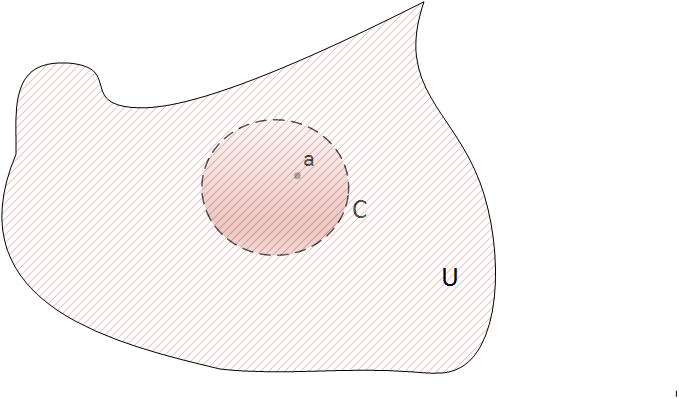# Cauchy's integral formula

### Basic concepts and principles

The integral formula of cauchy is so important that we reserve a complete section dedicated to it.Given f, a holomorphic function in an open set U and given a one point inside of U, then f is holomorphic in a disk surrounding point a. If C is the boundary of the disk then it is true the following
$$f(a) =\frac{1}{2\pi i}\ \int_{C} \frac{f(z)}{z-a}dz$$
This very important formula expresses the value of the function at a point inside the disk as function of some values in the boundary of the disk. Its proof, of which we will see an introduction is based on Cauchy's Theorem for a convex open:

### Proof of the Cauchy integral formula

Because f is holomorphic in all U, lets $a \in U$. For a we can

$\lim_{z \mapsto a} \frac{f(z)-f(a)}{z-a} = f'(a)$

So, it must to exists any $\epsilon > 0$ for wich if $|z-a| < \delta$

$\frac{f(z)-f(a)}{z-a} - f'(a) < \epsilon$

Then function given by

$g(z)=\left\{\begin{matrix} \frac{f(z)-f(a)}{z-a} & z\ne a\\ f'(a) & z=a \end{matrix}\right.$

Is continuous in all U and holomorphic in U-{a}.

Its line integral in every path that surrounds point a will be zero since Theorem3 of section complex integration, then

$\int_{C} g(z) dz = \int_{D(a, \epsilon)} g(z) dz$

Because can we take a radius as small as we want and and |g| is continuous into a compact set then it is bounded. Lets M be a bound of g, we have

$\int_{D(a, \epsilon)} |g(z)| dz \le M 2\pi \epsilon \underset{\epsilon \rightarrow 0}{\rightarrow} 0$

We can take for f

$\int_{C} \frac{f(z)}{z-a} dz = \int_{C} \frac{f(z)-f(a)}{z-a} dz + \int_{C} \frac{f(a)}{z-a} = 0 + 2\pi if(a)$

What is what we wanted to proof.

### Extended theory

The integral formula of Cauchy is also notable because it has important consequences, these are what make the branch of complex variable analysis unique into maths science

### Theorem 1: Infinite derivability of a holomorphic function

Given f, a holomorphic function in an open set U and given a, a point inside of U, then f has infinite derivatives in a and it is satisfied:
$$f^{(n)}(a) =\frac{n!}{2\pi i}\ \int_{C} \frac{f(z)}{(z-a)^{n+1}}dz$$
Observe that this formula contains the Cauchy's integral formula if n=0, with 0!=1.
The proof of this fact is based on fact of enter derivative sign into the integral sign

### Theorem 2: Cauchy's inequality

In the same conditions of the previous theorem, let M be a positive constant such that for all z of the curve C $|f(z)| \le M$, then it is fulfilled
$$|f^{n}(a)| \le \frac{M n!}{r^{n}}$$

The following theorem due to Liouville states that there can not be a bounded and holomorphic function in the whole complex plane except if it is constant

### Theorem 3: Liouville's Theorem

If f is holomorphic in the whole plane $\mathbb{C}$ and f is bounded, that is $|f(z)| \le M \forall z \in \mathbb{C}$ then f is constant.

The proof of Liouville's theorem is direct from Theorem 2 by taking increasingly larger radious.

### Theorem 4: Fundamental theorem of algebra

Lets $P(z) = a_{0} + a_{1}x + ... + a_{n}x^{n}$ a polynomial with complex coefficients of degree greater or equal than 1. Then P(z) has at least one root in $\mathbb{C}$.

Note that it actually has roots because$z_{0}$ is a root of P, then

$P(z) = (z-z_{0})( b_{0} + b_{1}x + ... + b_{n-1}x^{n-1}) = (z-z_{0}) Q(z)$.

Thus, Theorem 4 can be applied to Q(z). Operating by induction it is fulfilled that P(z) has complex roots

To prove Theorem 4, it is enough to consider

$f(z) = \frac{1}{P(z)}$.

If P(z) has not any root in $\mathbb{C}$ then f(z) is holomorphic in the whole plane $\mathbb{C}$, also f is bounded, by Liuoville's theorem f is constant, so P(z) would be constant which contradicts the hypothesis.

### Theorem 5: Principle of the maximum module

If f holomorphic on and into a region bounded by closed path C. Then the maximum of | f | occurs over C.
Note that the same can be applied to the minimum.

### Theorem 6: Medium Value theorem of Gauss

In the same conditions as Cauchy's integral formula, it is fulfilled

$f(a) = \frac{1}{2\pi} \int_{0}^{2 \pi} f(a+re^{i\theta}) d\theta$
The proof of this fact is easy, it is enough to observe that in the Cauchy's integral formula we parametrize C

$|z-a| = r \Rightarrow z= a + re^{i\theta}$.

Then Cauchy's integral formula becomes

$f(a)= \frac{1}{2\pi i} \int_{0}^{2 \pi} \frac {f(a+re^{i\theta}) ir e^{i\theta} }{r e^{i\theta}} d\theta = \frac{1}{2\pi} \int_{0}^{2 \pi} f(a+re^{i\theta}) d\theta$

What we wanted to proof.

## Was useful? want add anything?

Post here

### Post from other users

Post here